EENS 2120 Petrology Prof. Stephen A. Nelson Tulane University Thermodynamics and Metamorphism
 Equilibrium and Thermodynamics Although the stability relationships between various phases can be worked out using the experimental method, thermodynamics gives us a qualitative means of calculating the stabilities of various compounds or combinations of compounds (mineral assemblages).  We here give an introductory lesson in thermodynamics to help us better understand the relationships depicted on phase diagrams. The First Law of Thermodynamics states that "the internal energy, E, of an isolated system is constant". In a closed system, there cannot be a loss or gain of mass, but there can be a change in energy, dE.  This change in energy will be the difference between the heat, Q, gained or lost, and the work , W done by the system.  So, dE = dQ - dW                 (1) Work, W, is defined as force x distance.  Since Pressure, P, is defined as Force/surface area, Force = P x surface area, and thus W = P x surface area x distance = P x V, where V is volume.  If the work is done at constant pressure, then W = PdV.  Substitution of this relationship into (1) yields: dE = dQ - PdV                 (2) This is a restatement of the first law of thermodynamics.  The Second Law of Thermodynamics states that the change in heat energy of the system is related to the amount of disorder in the system.  Entropy is a measure of disorder, and so at constant Temperature and Pressure: dQ = TdS Thus, substituting into (2) we get: dE = TdS - PdV              (3) The Gibbs Free Energy, G, is defined as the energy in excess of the internal energy as follows: G = E + PV - TS                         (4) Differentiating this we get: dG = dE +VdP + PdV - TdS - SdT
 Substituting (3) into this equation then gives: dG = TdS - PdV + VdP + PdV - SdT - TdS  or dG = VdP - SdT     (5) For a system in equilibrium at constant P and T,  dG = 0.
 If we differentiate equation (5) with respect to P at constant T, the result is:(6)           and if we differentiate equation (5) with respect to T at constant P we get: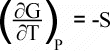(7) Equation (6) tells us that phases with small volume are favored at higher pressure, and equation (7) tells us that phases with high entropy (high disorder) are favored at higher temperature. Equation (5) tells us that the Gibbs Free Energy is a function of P and T.  We can see this with reference to the diagram below, which shows diagrammatically how G, T, and P are related in a system that contains two possible phases, A and B.

 In the diagram, phase A has a steeply sloping free energy surface.  Phase B has a more gently sloping surface.  Where the two surfaces intersect, Phase A is in equilibrium with phase B, and GA = GB.   Next we look at 3 cross-sections through this figure.  In the first, we look a section of G versus T at constant P, such as along the front face of the figure to the right.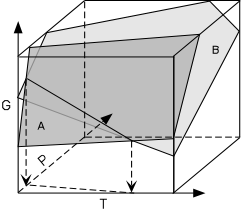In this section at constant P we see that at temperatures below TE phase A has the lowest Gibbs Free Energy, G.  At these temperatures, phase A is stable and phase B is not stable because it has a higher free energy.  Note that at TE the free energy of the phase A, GA, is the same as the free energy of phase B, GB.  At temperatures greater than TE phase B has a lower free energy than phase A, and thus phase B is stable.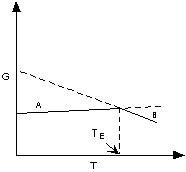Next, we look at a section of G versus P at constant T.  In this section, GA = GB at PE.  At pressures greater than PE phase B is stable because it has a lower G than phase A.  At pressures less than PE phase A is stable because it has a lower G than phase B.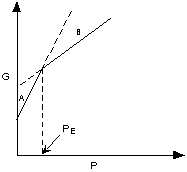Finally, we look at a cross-section across the bottom of the first figure.  Here we project the line of intersection of the Free Energy surfaces onto the P - T plane.  Along the line of intersection of the surfaces GA = GB.  The line separates two fields, one at low P in which A is the stable phase and one at higher P in which phase B is stable.  This is a classic P-T phase diagram.  The line represents all values of P and T where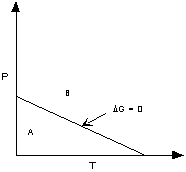Phase A and Phase B are in equilibrium or where GA = GB.  If the chemical reaction that occurs on this line is:  A <=> B    then we can write: ΔG = GB - GA = 0 Note that ΔG is defined as Gproducts - Greactants for the chemical reaction as written above. Taking a similar approach, we can rewrite equation (5), above, as: dΔG = ΔVdP - ΔSdT            (8) Where in general,  ΔG = the change in Gibbs Free Energy of the reaction =  ΣGproducts - ΣGreactants ΔS = the change in entropy of the reaction =  ΣSproducts - ΣSreactants and  ΔV = the change in volume of the reaction =  ΣVproducts - ΣVreactants At equilibrium, as we have just seen, ΔG = 0, so from equation (8) 0 = ΔVdP - ΔSdT Rearranging this equation yields( 9)

 This relation is known as the Clausius - Clapeyron Equation.  It is important  because it tells us the slope of the equilibrium boundary or reaction boundary on a Pressure versus Temperature phase diagram.

 We next look at two cases of chemical reactions.  In the first case, the chemical reaction is between only solid phases.  In the second case a fluid or gas is involved as one of the products of the reaction.
 Solid - Solid Reactions A solid-solid reaction only involves the solid phases as both reactants and products,  with no fluid phases showing up in the chemical reaction.  Most solid-solid reactions appear as straight lines on Pressure-Temperature diagrams.  The reason for this comes from the Clausius-Clapeyron equation.  dP/dT = ΔS/ΔV where ΔS = the change in entropy of the reaction =  ΣSproducts - ΣSreactants and ΔV = the change in volume of the reacti0on =  ΣVproducts - ΣVreactants In general, both the entropy, S, and the Volume, V of any phase varies with temperature and pressure.  As temperature increases, both S and V tend to increase (things become more disorganized at high temperature, increasing the entropy and molecules vibrate more at high temperature, increasing the volume).  Similarly, both S and V tend to decrease with increasing pressure (less room to vibrate means better organization and lower volume). In addition, the change in volume and entropy at any given temperature and pressure tends to be small.  The net result of this is that for solid - solid reactions the effects of increasing temperature tend to be offset by the effects of increasing pressure, and thus dP/dT remains more or less constant.  A curve whose slope is constant is a straight line.
 Let's use these principles to analyze some solid - solid reactions, such as those in the Al2SiO5  phase diagram.  Note that for the solid-solid reaction Andalusite <=> Kyanite, dP/dT is positive.  This is what we expect, because the product kyanite, occurs on the low T side of the reaction boundary and should have a lower entropy, making ΔS negative.  Increasing the pressure causes a decrease in volume, so Kyanite should have lower volume than Andalusite and thus  ΔV is also negative. With both ΔS and ΔV megative, the slope of the boundary curve dP/dT is positive.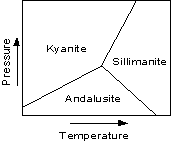For the reaction Kyanite <=> Sillimanite, the product Sillimanite occurs on the high T side of the boundary, and thus SKyanite < SSillimanite, so ΔS is positive.  Since Kyanite occurs on the high P side of the boundary curve, VKyanite < VSillimanite, so ΔV is also positive.  Thus, dP/dT is also positive. But, note that the reaction boundary for  Andalusite <=> Sillimanite has a negative slope on the diagram.  The product of the reaction, Sillimanite, has a smaller volume than the reactant Andalusite.  So, ΔV is negative.  But, Sillimanite occurs on the high temperature side of the reaction and thus has a higher entropy than andalusite.  Thus, since the reactant, andalusite, has a lower entropy then the product, sillimanite, ΔS is positive, making dP/dT negative.
 Devolatization Reactions Unlike sold-solid reactions, devolatilization reactions appear as curves on Pressure - Temperature diagrams.  to see why this is true let's analyze the simple dehydration reaction: A <=> B + H2O. For this reaction we can write: ΔS = SB + SH2O - SA = ΔSsolids +SH2O and ΔV = VB + VH2O - VA = ΔVsolids + VH2O
 Increasing temperature will generally cause DS to be positive, especially for this reaction in which a gas or fluid phase is produced, because gases always have a higher entropy (randomness) than solids.  At low pressure ΔVsolids will generally be negative. But at low pressure VH2O will be very large, because a fluid or gas will expand to fill space available.  Thus, dP/dT will be positive.  As the pressure increases, the fluid or gas will be more compressible than the solids, so the total ΔV will become increasingly smaller.  Thus, dP/dT will increase.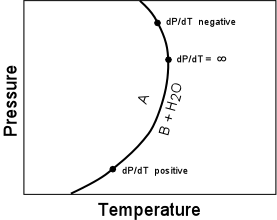Eventually because of the compressibility of the gas or fluid phase, ΔVsolids becomes equal to VH2O and thus ΔV of the reaction becomes 0, making dP/dT  infinite.  At pressures above this point, the fluid becomes so compressed that  VH2O gets smaller and  smaller, making ΔV for the reaction increasingly negative.  Thus, dP/dT becomes increasingly negative.
 Calculation of Reaction Boundaries Another relationship that is useful is: G = H - TS where G is the Gibbs Free Energy, H is the enthalpy, T is the absolute temperature in Kelvin, and S is the entropy. For a chemical reaction, we can rewrite this as: ΔG = ΔH - TΔS                     (10) where again: ΔG = the change in Free Energy of the reaction =  ΣGproducts - ΣGreactants ΔH = the change in Enthalpy of the reaction =  ΣHproducts - ΣHreactants ΔS = the change in Entropy of the reaction =  ΣSproducts - ΣSreactants In general ΔG, ΔH, ΔS, and ΔV are dependent of Pressure and Temperature, but at any given T & P: If ΔG < 0 (negative) the chemical reaction will be spontaneous and run to the right, If ΔG = 0 the reactants are in equilibrium with products, and if ΔG > 0 (positive) the reaction will run from right to left.   Temperature Dependence of G, H, and S As stated above, G, H, and S depend on Temperature and Pressure.  But, because G depends on H and S, it is usually more convenient to consider the temperature dependence of H and S, so that if we know H and S at any given temperature, we can calculate G.where Cp is the heat capacity at constant pressure.  The heat capacity is the amount of heat necessary to raise the temperature of the substance by 1o K. Thus: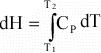or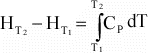If Cp is not a function of temperature, then further integration results in: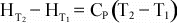(Note that in general, Cp is a function of temperature, and the known function of temperature could be inserted before integration, but this introduces complications that are beyond the scope of this course). Tables of thermodynamic data are usually tabulated at some known reference temperature and pressure, most commonly at a Temperature of 298 K, and Pressure of 1 bar ( = 0.1 MPa ~ 1 atm).  Thus, we if we need to know H at some temperature, T, other than 298 K, we can use the above equation to determine H at the new temperature: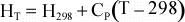For a reaction, the above equation can be rewritten as: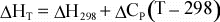(11) The temperature dependence of entropy, S, is given by:orAgain, if Cp is not a function of T, then integration results in:Or, since data are usually available at 298 K and 0.1MPa, for a reaction, this can be written as: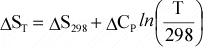(12)    Equation 10 can then be combined with equations 11 and 12 to give the dependence of ΔG on temperature: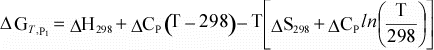We can simplify this even further if we assume that for a reaction, ΔCp = 0: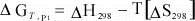(13) Thus, using the assumptions above, we can now calculate ΔG at our reference pressure, P1 at any temperature, if we know ΔH and ΔS at our reference temperature of 298 K.

Pressure Dependence of G and ΔG

From equation 6, above:or, for a reaction: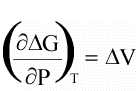Integrating this, results in: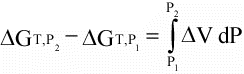If we assume that ΔV is not a function of pressure (in general this is a good assumption for solids, but not for liquids or gases, as we have seen above), then integration results in: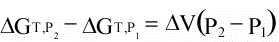(14)

Notice that the second term on the left in equation 14 is the same equation (13), above.  Thus, we can substitute equation 13 into equation 14, and rearrange to get our final expression for ΔG as a function of Pressure and Temperature:(15)

Again, however, we must remember the assumptions involved in using this equation.  The assumptions are that ΔCP = 0 and ΔV is not a function of pressure.

Calculation of Reaction Boundaries

If we have thermodynamic data for minerals involved in a chemical reaction, then we can use equation 15 to calculate reaction boundaries on a Pressure - Temperature diagram.  Let's consider the following chemical reaction:

NaAlSi3O8 <=> NaAlSi2O6 + SiO2

 Phase ΔH298, 0.1Mpa  (joules/mole) S298, 0.1Mpa (joules/Kmole) V298, 0.1Mpa (cm3/mole) albite -3921618.201 224.12 100.83 jadeite -3025118.24 133.574 60.034 quartz -908626.77 44.207 23.7

(Data from Berman, 1988)

For the reaction, at equilibrium, we can write:

ΔH298, 0.1Mpa = ΔHjadeite + ΔHquartz - ΔHalbite = -3025118.24 + (-908626.77) - (-3921618.201)

ΔS298, 0.1Mpa = Sjadeite + Squartz - Salbite = 133.574 + 44.207 - 224.12

ΔV298, 0.1Mpa = Vjadeite + Vquartz - Valbite = 60.034 + 23.7 - 100.83

Then, since at equilibrium, ΔG = 0, we can write:To be consistent we should have ΔV in units of m3/mole, in which case P will have units of Pa (Pascals).  But since our initial atmospheric pressure is 0.1 MPa, if we leave ΔV in units of cm3/mole, the results will come out with pressure units of MPa  (note that 103 MPa = 1GPa).

Plugging in the values of ΔH, ΔS, andΔV, as shown above, we can then plug in a value of T (in Kelvin) and solve for P (in MPa).

 The results should look something like the graph shown here, where Pressure has been converted to GPa and temperature is shown in oC. The dashed line in the graph shows the same boundary calculated using a more vigorous approach, that is taking into account the variation of ΔH with temperature and ΔV with pressure.Note that the reaction boundary is still a straight line, as we would expect from our analysis of solid-solid reactions and the Clapeyron equation, as discussed above.
 We next consider the more complicated case of a dehydration reaction.  The reaction we will consider is: KAl3Si3O10(OH)2 + SiO2 <=> KAlSi3O8 + Al2SiO5 + H2O       muscovite          quartz             kspar      sillimanite   fluid In this reaction, the Al2SiO5 mineral is shown as sillimanite, whereas in reality we would have to take into consideration that the reaction boundary may cross the stability fields of andalusite, sillimanite, and kyanite.  For simplicity sake, however, we will just consider sillimanite. Because this reaction involves a fluid or gas phase, our assumption about ΔV being a constant will more than likely not be true, since we know that fluids and gases are easily compressed with increasing pressure and expand with increasing temperature, much more so than do solid phases. As a first approximation, we note that for a gas phase, the ideal gas law states that: PV = nRT or V = nRT/P again, showing that the volume of the gas is a function of temperature and pressure.  Thus, substituting V from the ideal gas law into equation 6, above, results in: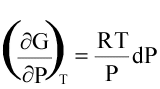Integrating this from P1 to P2 results in: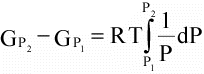orFor the gas phase we can let P1 be the reference pressure (0.1 MPa), and the values of G can be at the pressure, P, and temperature, T,  of the reaction, so that we can rewrite this as:(16) If, as we did before, we calculate ΔG for this reaction, including all phases including the gas as part of the ΔH and ΔS terms, including only the solids in the ΔV term, and expressing the variation of the volume of gas as a function of pressure, as shown above, then:     ΔH298, 0.1Mpa = ΔHkspar + ΔHsillimanite + ΔHH2O - (ΔHmuscovite + ΔHquartz)     ΔS298, 0.1Mpa = Skspar + Ssillimanite + SH2O - (Smuscovite + Squartz) ΔV298, 0.1Mpa = Vkspar + Vsillimanite - (Vmuscovite + Vquartz) = ΔVsolids Then, substituting these values along with equation (16) into equation (15),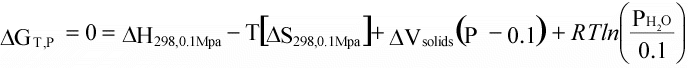If we assume that PH2O = P, then we can substitute to get:(17) This equation is difficult to separate so that T can solved for P or P solved for T.  With a spreadsheet, however, one can set up the equation so that for each T on can guess at a value of P until one is found that makes ΔG = 0.  One can then make a plot of P vs. T to show the reaction curve.
 Such a plot is shown here. Again, we should review the assumptions behind this solution.  We are assuming that ΔCp = 0, that ΔV is not a function of pressure, that sillimanite is the Al2SiO5 mineral produced in the reaction, that muscovite, kspar, quartz, and sillimanite are pure phases (not solid solutions), and that H2O behaves as an ideal gas and is the only gas present in the fluid phase.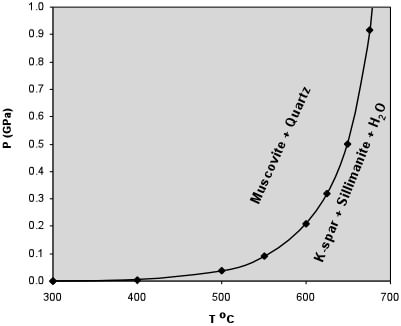Note how the reaction boundary is curved and how it asymptotically approaches the temperature axis at low temperature.  This is typical of dehydration and decarbonation reactions. The assumptions about the lack of solid solution in the solids is pretty good to for these solids to a first approximation (with the possible exception of k-spar), but in the earth, it is likely that the fluid phase is not pure H2O, and is more likely a mixture of H2O and CO2. We can take this into account by substituting the partial pressure, pH2O of H2O in equation (17).  pH2O = XH2OP where XH2O = the mole fraction of H2O in the fluid or gas phase.  Equation (17) then becomes: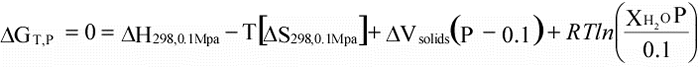Before looking at the results of this equation, let's reason out what effects we should see.  If we look at the reaction: KAl3Si3O10(OH)2 + SiO2 <=> KAlSi3O8 + Al2SiO5 + H2O       muscovite          quartz             kspar      sillimanite   fluid we should be able to see that reducing the amount of H2O on the right-hand side of the equation should make the reaction run from left to right, thus decreasing the stability of muscovite + quartz.
 If we now solve the equation, again by setting up a spreadsheet so that we can take guesses at the value of P necessary to reduce ΔG to 0 at any given T, we get the graph shown here.  It is notable that our reasoning about decreasing the stability of muscovite + quartz was correct.Recall that we said that most of the phases with the exception of the fluid phase and possibly kspar in this reaction are pure.  Let's now consider what the effect would be on the reaction curve if kspar was actually a solid solution between pure KAlSi3O8 and NaAlSi3O8, which of course it is in nature.Again, looking at the reaction: KAl3Si3O10(OH)2 + SiO2 <=> KAlSi3O8 + Al2SiO5 + H2O       muscovite          quartz             kspar      sillimanite   fluid we should be able to see that reducing the concentration of KAlSi3O8 in the kspar solid solution should have a similar effect as reducing the concentration of H2O in the fluid phase.  That is the stability curve would move to lower temperature at any given pressure, thus reducing the stability of muscovite + quartz. By similar reasoning, if one of the reactants, say muscovite were not pure muscovite, but instead was a solid solution, the reaction would begin to run from right to left, thus increasing the stability of the products and moving the reaction boundary to higher temperature at any given pressure. Unfortunately, we do not have time in this course to explore how impure phases are taken care of in the thermodynamic treatment.  The basic principals of the thermodynamic treatment presented here allow metamorphic petrologists to work out reactions that can serve as geothermometers (to determine temperature) and geobarometers (to determine pressure), and thus determine where in the earth metamorphism took place in a given suite of metamorphic rocks.
 Reaction Rates (Kinetics) Thermodynamics can tell us what mineral phases are in equilibrium at a specific temperature and pressure, but does not tell us anything about the rates at which chemical equilibrium is achieved.   During prograde metamorphism, temperatures are generally increasing, and rates of chemical reactions tend to increase with increasing temperature.  Thus, during prograde metamorphism  there is usually ample time for equilibrium to occur.  But, as these minerals are brought back to the pressure temperature conditions present at the Earth's surface, temperature will be decreasing.  The rates of the reactions will thus be much slower, and phases stable at high pressure and temperature will often be preserved when we find the rocks exposed at the surface.  Thus, it is common to find metastable phases, i.e. phases that are not stable at the surface, but represent equilibrium at some higher temperature and pressure.
 Examples of questions on this material that could be asked on an exam What is the Clausius - Clapeyron Equation and what does it tell us? Give an example of a dehydration reaciton and a decarbonation reaction. Why are solid-solid reaction boundaries on Pressure vs. Temperature diagrams straight lines and  dehydration or decarbonation reaction boundaries curved lines. In order for all phases in a chemical reaction to be at equilibrium, what must be true of the Gibbs free energy change of the reaction (ΔG)? Which thermodynamic properties of minerals must one know in order to calculate the reaction boundary for a chemical reaction? Return to EENS 2120 Home Page